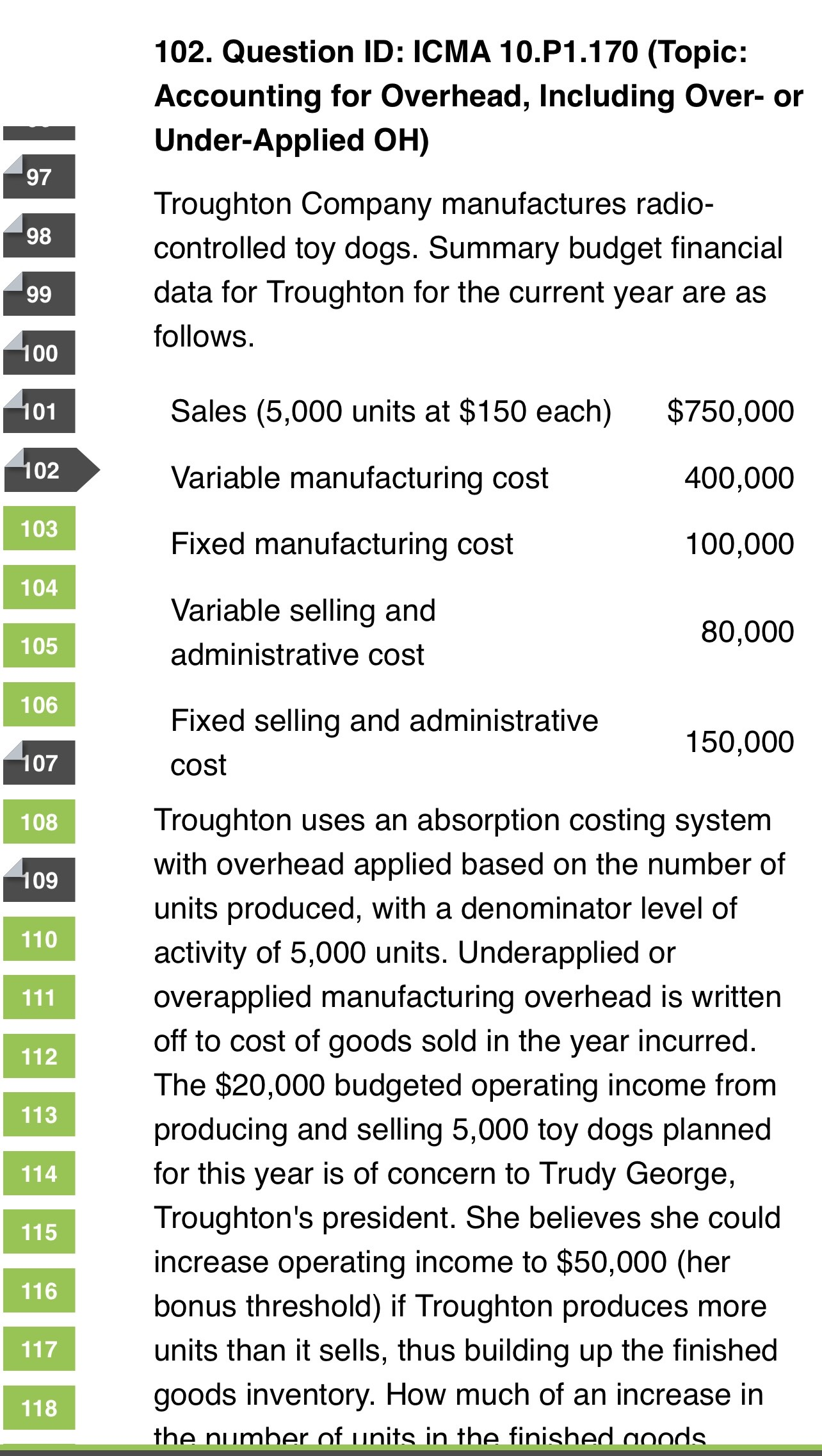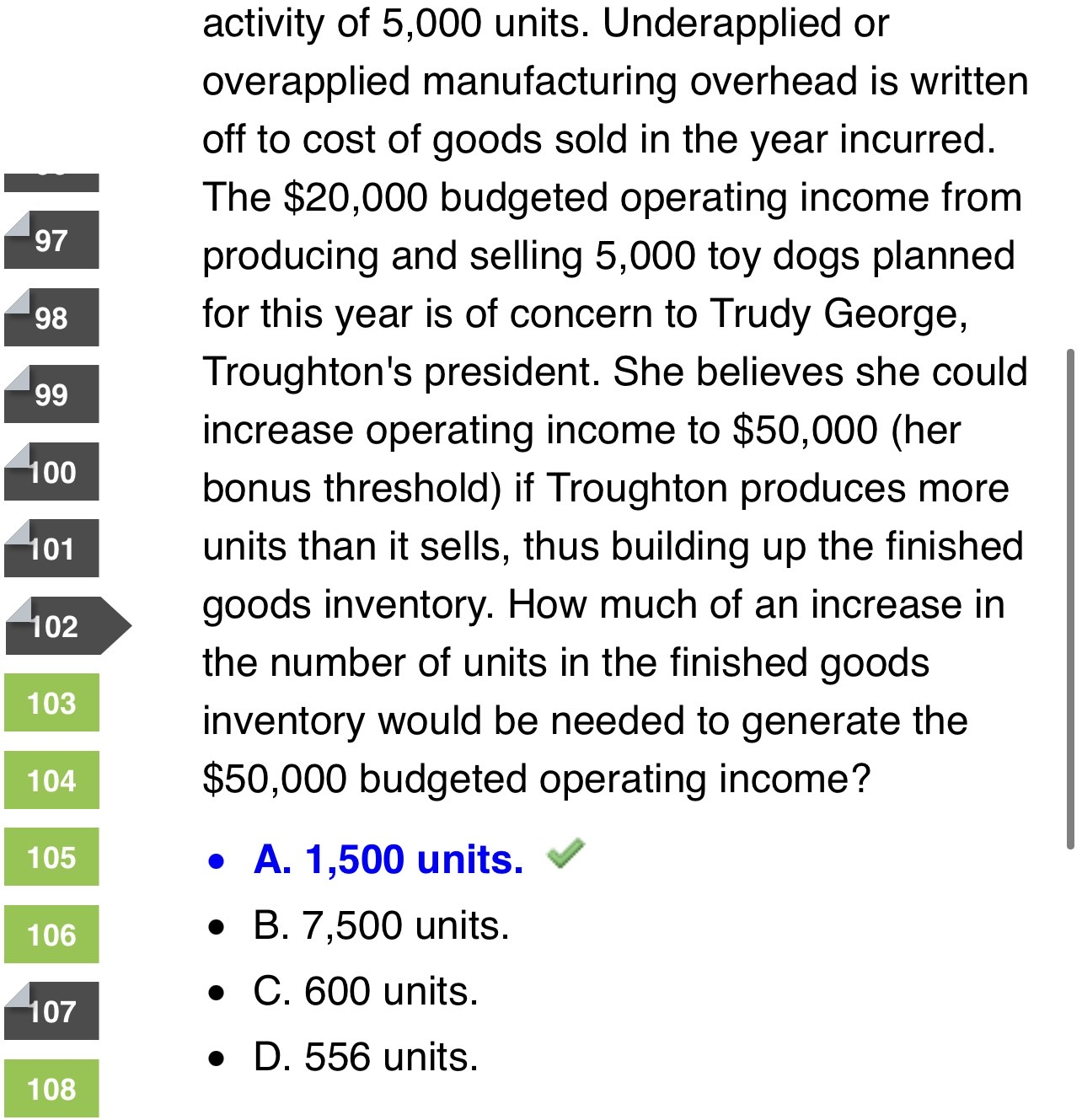# CMA Study Group

View Only

## Question section D -CMA PART1#### Mohamed Adel Kotb23 days ago• #### 1.  Question section D -CMA PART1

Posted 24 days ago
Hello everyone ,
Can someone help me understand the solution to this question?
Why was only the fixed manufacturing  costs charged to the inventory (FG)?• #### 2.  RE: Question section D -CMA PART1

Posted 23 days ago
"if the company produces more units than it sells, thus building up the finished goods inventory".
and on of the main differences between Direct costing and Apsorbtion costing is how to treat the fixed cost.
The question mean that under this costing method he will produce more to spread the fixed cost over more unit which will reduce fixed cost per unit
in this case Fixed cost per unit is 100,000/5,000 unit = \$20 , and we need to save \$30,000 from increasing the production thus we need 1,500 unit (30,000/20) must be produced and moved to ending inventory

regarding your question why only fixed cost:
under absorption costing, fixed costs can be piled up in ending inventory

hope it become clear now and if not you are welcome to ask for further clarification

------------------------------
Other
Dammam
Saudi Arabia
------------------------------

• #### 3.  RE: Question section D -CMA PART1

Posted 23 days ago

Hi
This question is based on the drawback of Absorption costing or Full costing.

The Concerned manager wants to increase the profit from 20000  to 50000, and she wants to do that not by increasing sales  or increasing sales price.
rather she wants to increase the profit by taking the advantages of  Absorption costing concept  i.e to divert some amount of Fixed manufacturing OH to B/S  with the closing Finished goods unit.

If u will do the calculation of allocation of Oh u will find the following
1. Total OH need to be allocated 100000

2.Denominator level of activity of 5,000 units.

Hence per unit FMOH allocation =  100000/5000=20 , right?
He wants to increase the income from 20000 to 50000, means increment = 30000(50000-20000)
From this we can assume , that she wants to divert 30000 from the  COGS to  BS

so number of units more required   =  30000/20= 1500 units.

------------------------------
------------------------------

• #### 4.  RE: Question section D -CMA PART1

Posted 23 days ago
only cost that will increase the value of inventory is fixed manufacturing cost
the objective of the manager in the given question is to increase the profit from \$ 20000 to \$ 50000/- ( increase by \$ 30000/-)
We know...ONLY fixed manufacturing costs are absorbed based on planned production unit overhead absorption rate
it is given in the question the production & sales are same quantity 5000 units...so Fixed Manuf overhead absorption rate is 100000/5000 = 20/- per unit
So when the manager decided to produce 1500 more units (that is total 6500 units and sells only 5000 units )

your PNL will be as follows :

Sales   = 5000 units x 150 = 750,000
COGS = 5000 units x  100  = (500,000) ( variable 80 + 20 fixed manufacturing overhead)
Over absorbed/over applied = 30,000 ( gain ...refer note 1 below)
Gross Margin             =  280000
Less :
Variable selling               (80000)